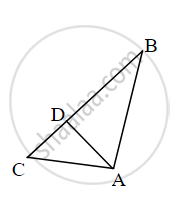# From given figure, In ∆ABC, AD ⊥ BC, then prove that AB2 + CD2 = BD2 + AC2 by completing activity.Activity: From given figure, In ∆ACD, By pythagoras theorem AC2 = AD2 + □ ∴ AD2 = AC2 – CD2 ..... - Geometry

Sum

From given figure, In ∆ABC, AD ⊥ BC, then prove that AB2 + CD2 = BD2 + AC2 by completing activity.Activity: From given figure, In ∆ACD, By pythagoras theorem

AC2 = AD2 + square

∴ AD2 = AC2 – CD2    ......(I)

Also, In ∆ABD, by pythagoras theorem,

AB2 = square + BD2

∴ AD2 = AB2 – BD2    ......(II)

∴ square − BD2 = AC2 − square

∴ AB2 + CD2 = AC2+ BD2

#### Solution

From given figure, in ∆ACD, By pythagoras theorem

∴ AD2 = AC2 – CD2    ......(I)

Also, In ∆ABD, by pythagoras theorem,

∴ AD2 = AB2 – BD2    ......(II)

AB2 − BD2 = AC2CD2     ......[From (i) and (ii)]

∴ AB2 + CD2 = AC2+ BD2

Concept: Similarity in Right Angled Triangles
Is there an error in this question or solution?

Share